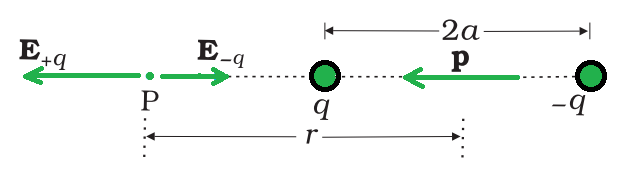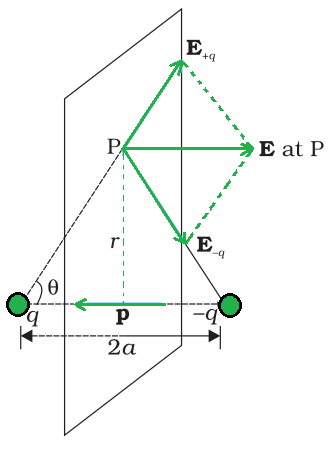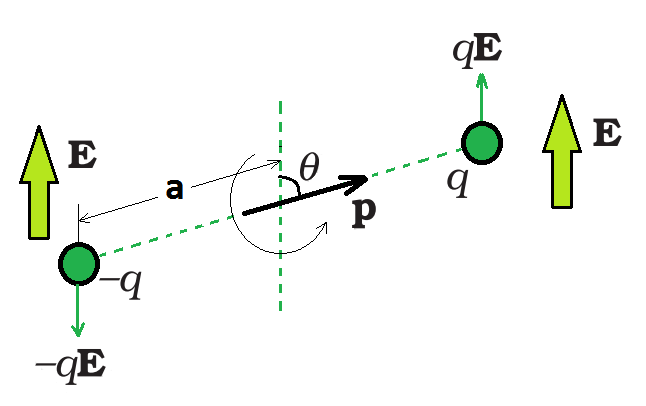Related Articles

# Electric Dipole

• Last Updated : 14 Jul, 2021

Electric charge is a fundamental property of matter that controls how an electric or magnetic field affects elementary particles. Electric charge exists in discrete natural units that cannot be generated or destroyed. Positive and negative charges are the two types of electric charges. When two items with an excess of one type of charge are near enough together, they repel each other. When two positively charged and negatively charged objects are in close contact, they attract one other.

Many fundamental, or subatomic, particles of matter share the property of electric charge. Protons have a positive charge, whereas electrons have a negative charge. Neutrons, on the other hand, are uncharged that is neutral particles. The negative charge of each electron has the same magnitude as the positive charge of each proton. The charge of an electron or proton, which is a fundamental physical constant, is measured in natural units.

Attention reader! All those who say programming isn't for kids, just haven't met the right mentors yet. Join the  Demo Class for First Step to Coding Course, specifically designed for students of class 8 to 12.

The students will get to learn more about the world of programming in these free classes which will definitely help them in making a wise career choice in the future.

### Electric Dipole

A pair of equal and opposite point charges q and –q separated by a distance 2a form an electric dipole and the electric dipole moment (p) is the product of the charge and the space between the charges (2a), is used to determine the strength of an electric dipole.

A direction in space is defined by the line joining the two charges. The direction from –q to q is commonly referred to as the dipole’s direction. The center of the dipole is the location of the middle point of –q and q.

The electric dipole’s overall charge is definitely zero. This does not imply that the electric dipole’s field is zero. Because the charges q and –q are separated by a certain distance, the electric fields produced by them do not exactly cancel out when put together. The fields attributable to q and –q almost cancel out over distances much greater than the spacing of the two charges producing a dipole (r >> 2a). As a result, the electric field produced by a dipole decays faster than 1/r2 (the dependence of the field due to a single charge q on r).

These qualitative ideas are borne out by the explicit calculation as follows:

• The field of an electric dipole

Coulomb’s law and the superposition principle may be used to calculate the electric field of a pair of charges (–q and q) at any point in space. For the following two scenarios, the results are simple and clear,

1. When the point is on the dipole axis,
2. When it is on the equatorial plane of the dipole, i.e. on a plane perpendicular to the dipole axis through its center.

By applying the parallelogram law of vectors, the electric field at any general point P is determined by summing the electric fields E–q due to the charge –q and E+q due to the charge q.

•  For points on the axisThe electric field of a dipole at a point on the axis.

Suppose the point P be at distance r from the center of the dipole on the side of the charge q. Then  electric field E–q  due to the charge –q can be expressed as,whereis the unit vector along the dipole axis that is from –q to q. Similarly, Then electric field E+q due to the charge +q can be expressed as,Now, the total field at P can be calculated by adding the electric fields E–q due to the charge –q and E+q due to the charge +q and can be expressed as,For r >> a, the above expression can be written as,• For points on the equatorial planeThe electric field of a dipole at a point on the equatorial plane of the dipole. p is the dipole moment vector of magnitude p = q×2a and directed from –q to q.

Then, the electric field E+q due to the charge +q can be expressed as,Similarly, then the electric field E-q due to the charge –q can be expressed as,It is observed that the electric fields E–q due to the charge –q and E+q due to the charge +q and are equal. The E+q and E–q directions are displayed in the above-given figure. The components normal to the dipole axis clearly cancel out. Along the dipole axis, the components add up. The entire electric field is in the opposite direction of.

The above expression can be added as,At large distances (r >> a), the above expression can be written as,At great distances, it is evident in both cases that the dipole field does not involve q and a separately; it is dependent on the product qa. This hints at the meaning of the dipole moment. The dipole moment of an electric dipole is a vector quantity and it is symbol is p  is defined byThe electric field of a dipole at large distances (r >> a) assumes simple shapes in terms of p:

• At a point on the dipole axis:• At a point on the equatorial plane:It’s worth noting that the dipole field at great distances decreases off as 1/r 3 rather than 1/r 2. Furthermore, the dipole field’s amplitude and direction are dependent not only on the distance r but also on the angle formed by the position vector r and the dipole moment p.

### Physical Significance of Dipoles

The centers of the positive and negative charge are located at the same location in most molecules. As a result, their dipole moment is nil. CO2 and CH4 are examples of this class of molecule. When an electric field is applied, they form a dipole moment. However, the centers of negative and positive charges may not always coincide in particular compounds. As a result, even in the absence of an electric field, they have a persistent electric dipole moment. Polar molecules are the name for these types of molecules. H2O molecules, for example, are an example of this type.

### Dipole in a Uniform External FiledDipole in a uniform electric field.

Suppose a permanent dipole in a uniform external field E with a dipole moment of p. On q, there is a force qE and on –q, there is a force –qE. Because E is uniform, the net force on the dipole is zero. Due to the separation of the charges, the forces operate at various places, causing a torque on the dipole. The torque (couple) is independent of the origin when the net force is zero. Its amplitude is equal to the sum of the magnitudes of the two antiparallel forces multiplied by the couple’s arm (perpendicular distance between the two antiparallel forces). The magnitude of torque can be expressed as,

τ = q E × 2 a sinθ

or τ  = 2 q a E sinθ

Its direction is perpendicular to the plane of the paper, coming out of it. The magnitude of p × E is also pE sinθ and its direction is normal to the paper, coming out of it.

τ = = p × E

The dipole will tend to align with the field E as a result of this torque. The torque is 0 when p is aligned with E.

If the field is not uniform, the net force will undoubtedly be greater than zero. Additionally, like before, there will be torque on the system. Because the general case is complicated, consider the simpler cases when p is parallel to E or antiparallel to E. The net torque is zero in both cases, but there is a net force on the dipole if E is not uniform.

### Sample Problems

Problem 1: Define the term electric dipole moment of a dipole. State it’s S.I. unit.

Solution:

A pair of equal and opposite point charges q and –q separated by a distance 2a form an electric dipole and the electric dipole moment (p) is the product of the charge and the space between the charges (2a), is used to determine the strength of an electric dipole. Its SI unit is Cm.or

|P|=q|2a|

Problem 2: In which orientation, a dipole placed in a uniform electric field is in a stable, unstable equilibrium?

Solution:

A dipole is placed parallel to the electric field for stable balance and a dipole is placed antiparallel to the electric field for unstable equilibrium.

If the field is not uniform, the net force will undoubtedly be greater than zero. Additionally, like before, there will be torque on the system. Because the general case is complicated, consider the simpler cases when p is parallel to E or antiparallel to E. The net torque is zero in both cases, but there is a net force on the dipole if E is not uniform.

Problem 3: Why are electric field lines perpendicular at a point on an equipotential surface of a conductor?

Solution:

There would be a non-zero component along the surface if the electric field lines were not normal to the equipotential surface. Work would be required to move a unit test charge against the component of the field’s direction, indicating that this surface cannot be an equipotential surface. As a result, electric field lines are perpendicular at a point on a conductor’s equipotential surface.

Problem 4: Given a uniform electric field, find the flux of this field through a square of side 20 cm, whose plane is parallel to the y-z plane. What would be the flux through the same square, if the plane makes an angle of 30° with the x-axis?

Solution:

Given,

Electric field isA = 10 × 10 × 10-4m2,

Flux (ϕ) = EA cos θ

Case 1,

θ = 0°,

or cos 0° = 1

Therefore,  Flux, ϕ= (5 × 103) × (10 × 10 × 10-4) cos 0°

ϕ = 50 Nm2C-1

Case 2,

Angle of square plane with x-axis = 30°

Hence, the angle will be (90° – 30°) = 60°

ϕ = EA cos θ

ϕ = (5 × 103) × (10 × 10 × 10-4) × cos 60°

ϕ = 50 × 1/2

ϕ = 25 Nm2C-1

Problem 5: Define the term ‘electric flux’. Write it’s S.I. units. What is the flux due to electric field through a square of side 10 cm, when it is held normal too if?

Solution:

The total number of lines of force moving through an area in an electric field is known as electric flux. It’s represented by the symbol ϕ. It’s a number with a scalar value. Its S.I unit is Nm2 C-1 or Vm.

It is expressed as,Given,

Electric field is 3×103 N/C.

Area is (10/100)×(10/100) m2 = 10-2 m2

θ = 0°,

or

cos 0° = 1

The expression for the flux can be written as,

ϕ = EA cos θ

Therefore, Flux, ϕ= (3 × 103) × (10-2) cos 0°

ϕ = 30 Nm2C-1

Problem 6: Why do the electric field lines not form closed loops?

Solution:

Because the direction of an electric field is from positive to negative charge, it does not form closed loops. As a result, a line of force can be seen as starting with a positive charge and terminating with a negative charge.

Problem 7: Is the electric field due to a charge configuration with total charge zero, necessarily zero? Justify.

Solution:

No, it is not necessarily zero. If the electric field due to a charge configuration with total charge is zero because the electric field due to an electric dipole is non-zero.

My Personal Notes arrow_drop_up